# MultilayerPerceptron: A simple multilayer neural network

Implementation of a multilayer perceptron, a feedforward artificial neural network.

from mlxtend.classifier import MultiLayerPerceptron

## Overview

Although the code is fully working and can be used for common classification tasks, this implementation is not geared towards efficiency but clarity – the original code was written for demonstration purposes.

### Basic Architecture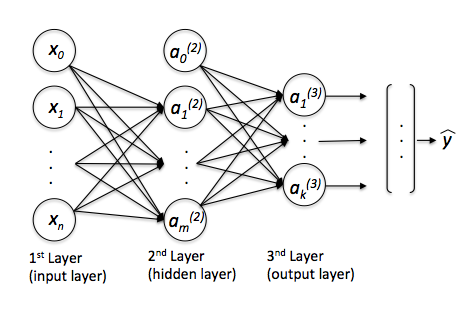The neurons $x_0$ and $a_0$ represent the bias units ($x_0=1$, $a_0=1$).

The $i$th superscript denotes the $i$th layer, and the jth subscripts stands for the index of the respective unit. For example, $a_{1}^{(2)}$ refers to the first activation unit after the bias unit (i.e., 2nd activation unit) in the 2nd layer (here: the hidden layer)

Each layer $(l)$ in a multi-layer perceptron, a directed graph, is fully connected to the next layer $(l+1)$. We write the weight coefficient that connects the $k$th unit in the $l$th layer to the $j$th unit in layer $l+1$ as $w^{(l)}_{j, k}$.

For example, the weight coefficient that connects the units

$a_0^{(2)} \rightarrow a_1^{(3)}$

would be written as $w_{1,0}^{(2)}$.

### Activation

In the current implementation, the activations of the hidden layer(s) are computed via the logistic (sigmoid) function $\phi(z) = \frac{1}{1 + e^{-z}}.$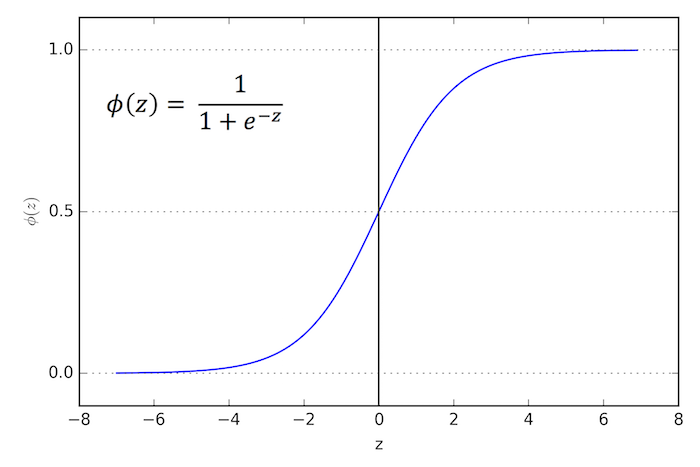(For more details on the logistic function, please see classifier.LogisticRegression; a general overview of different activation function can be found here.)

Furthermore, the MLP uses the softmax function in the output layer, For more details on the logistic function, please see classifier.SoftmaxRegression.

## Example 1 - Classifying Iris Flowers

Load 2 features from Iris (petal length and petal width) for visualization purposes:

from mlxtend.data import iris_data
X, y = iris_data()
X = X[:, [0, 3]]

# standardize training data
X_std = (X - X.mean(axis=0)) / X.std(axis=0)


Train neural network for 3 output flower classes ('Setosa', 'Versicolor', 'Virginica'), regular gradient decent (minibatches=1), 30 hidden units, and no regularization.

Setting the minibatches to 1 will result in gradient descent training; please see Gradient Descent vs. Stochastic Gradient Descent for details.

from mlxtend.classifier import MultiLayerPerceptron as MLP

nn1 = MLP(hidden_layers=,
l2=0.00,
l1=0.0,
epochs=150,
eta=0.05,
momentum=0.1,
decrease_const=0.0,
minibatches=1,
random_seed=1,
print_progress=3)

nn1 = nn1.fit(X_std, y)

Iteration: 150/150 | Cost 0.06 | Elapsed: 0:00:00 | ETA: 0:00:00

from mlxtend.plotting import plot_decision_regions
import matplotlib.pyplot as plt

fig = plot_decision_regions(X=X_std, y=y, clf=nn1, legend=2)
plt.title('Multi-layer Perceptron w. 1 hidden layer (logistic sigmoid)')
plt.show()import matplotlib.pyplot as plt
plt.plot(range(len(nn1.cost_)), nn1.cost_)
plt.ylabel('Cost')
plt.xlabel('Epochs')
plt.show()print('Accuracy: %.2f%%' % (100 * nn1.score(X_std, y)))

Accuracy: 96.67%


Setting minibatches to n_samples will result in stochastic gradient descent training; please see Gradient Descent vs. Stochastic Gradient Descent for details.

nn2 = MLP(hidden_layers=,
l2=0.00,
l1=0.0,
epochs=5,
eta=0.005,
momentum=0.1,
decrease_const=0.0,
minibatches=len(y),
random_seed=1,
print_progress=3)

nn2.fit(X_std, y)

plt.plot(range(len(nn2.cost_)), nn2.cost_)
plt.ylabel('Cost')
plt.xlabel('Epochs')
plt.show()

Iteration: 5/5 | Cost 0.11 | Elapsed: 00:00:00 | ETA: 00:00:00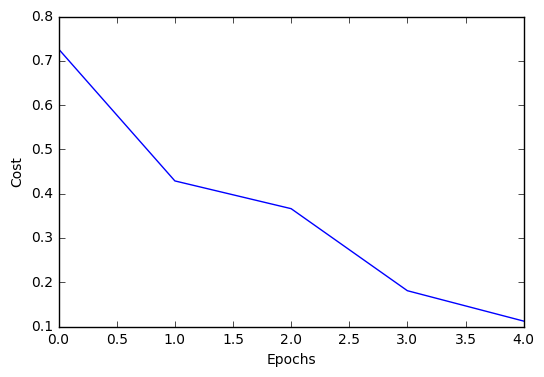Continue the training for 25 epochs...

nn2.epochs = 25
nn2 = nn2.fit(X_std, y)

Iteration: 25/25 | Cost 0.07 | Elapsed: 0:00:00 | ETA: 0:00:00

plt.plot(range(len(nn2.cost_)), nn2.cost_)
plt.ylabel('Cost')
plt.xlabel('Epochs')
plt.show()## Example 2 - Classifying Handwritten Digits from a 10% MNIST Subset

Load a 5000-sample subset of the MNIST dataset (please see data.loadlocal_mnist if you want to download and read in the complete MNIST dataset).

from mlxtend.data import mnist_data
from mlxtend.preprocessing import shuffle_arrays_unison

X, y = mnist_data()
X, y = shuffle_arrays_unison((X, y), random_seed=1)
X_train, y_train = X[:500], y[:500]
X_test, y_test = X[500:], y[500:]


Visualize a sample from the MNIST dataset to check if it was loaded correctly:

import matplotlib.pyplot as plt

def plot_digit(X, y, idx):
img = X[idx].reshape(28,28)
plt.imshow(img, cmap='Greys',  interpolation='nearest')
plt.title('true label: %d' % y[idx])
plt.show()

plot_digit(X, y, 3500)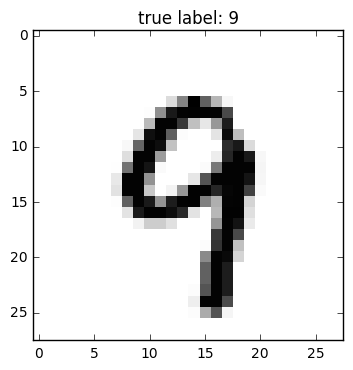Standardize pixel values:

import numpy as np
from mlxtend.preprocessing import standardize

X_train_std, params = standardize(X_train,
columns=range(X_train.shape),
return_params=True)

X_test_std = standardize(X_test,
columns=range(X_test.shape),
params=params)


Initialize the neural network to recognize the 10 different digits (0-10) using 300 epochs and mini-batch learning.

nn1 = MLP(hidden_layers=,
l2=0.00,
l1=0.0,
epochs=100,
eta=0.005,
momentum=0.0,
decrease_const=0.0,
minibatches=100,
random_seed=1,
print_progress=3)


Learn the features while printing the progress to get an idea about how long it may take.

import matplotlib.pyplot as plt

nn1.fit(X_train_std, y_train)

plt.plot(range(len(nn1.cost_)), nn1.cost_)
plt.ylabel('Cost')
plt.xlabel('Epochs')
plt.show()

Iteration: 100/100 | Cost 0.01 | Elapsed: 0:00:17 | ETA: 0:00:00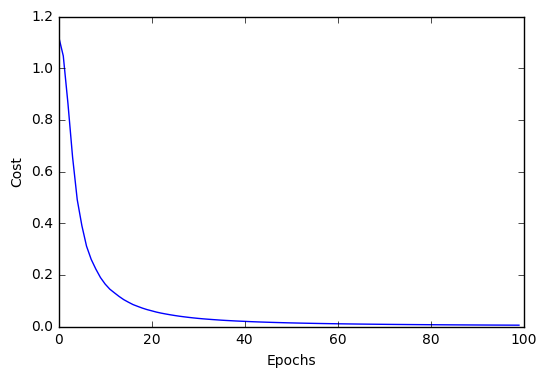print('Train Accuracy: %.2f%%' % (100 * nn1.score(X_train_std, y_train)))
print('Test Accuracy: %.2f%%' % (100 * nn1.score(X_test_std, y_test)))

Train Accuracy: 100.00%
Test Accuracy: 84.62%


Please note that this neural network has been trained on only 10% of the MNIST data for technical demonstration purposes, hence, the lousy predictive performance.

# API

MultiLayerPerceptron(eta=0.5, epochs=50, hidden_layers=, n_classes=None, momentum=0.0, l1=0.0, l2=0.0, dropout=1.0, decrease_const=0.0, minibatches=1, random_seed=None, print_progress=0)

Multi-layer perceptron classifier with logistic sigmoid activations

Parameters

• eta : float (default: 0.5)

Learning rate (between 0.0 and 1.0)

• epochs : int (default: 50)

Passes over the training dataset. Prior to each epoch, the dataset is shuffled if minibatches > 1 to prevent cycles in stochastic gradient descent.

• hidden_layers : list (default: )

Number of units per hidden layer. By default 50 units in the first hidden layer. At the moment only 1 hidden layer is supported

• n_classes : int (default: None)

A positive integer to declare the number of class labels if not all class labels are present in a partial training set. Gets the number of class labels automatically if None.

• l1 : float (default: 0.0)

L1 regularization strength

• l2 : float (default: 0.0)

L2 regularization strength

• momentum : float (default: 0.0)

Momentum constant. Factor multiplied with the gradient of the previous epoch t-1 to improve learning speed w(t) := w(t) - (grad(t) + momentum * grad(t-1))

• decrease_const : float (default: 0.0)

Decrease constant. Shrinks the learning rate after each epoch via eta / (1 + epoch*decrease_const)

• minibatches : int (default: 1)

Divide the training data into k minibatches for accelerated stochastic gradient descent learning. Gradient Descent Learning if minibatches = 1 Stochastic Gradient Descent learning if minibatches = len(y) Minibatch learning if minibatches > 1

• random_seed : int (default: None)

Set random state for shuffling and initializing the weights.

• print_progress : int (default: 0)

Prints progress in fitting to stderr. 0: No output 1: Epochs elapsed and cost 2: 1 plus time elapsed 3: 2 plus estimated time until completion

Attributes

• w_ : 2d-array, shape=[n_features, n_classes]

Weights after fitting.

• b_ : 1D-array, shape=[n_classes]

Bias units after fitting.

• cost_ : list

List of floats; the mean categorical cross entropy cost after each epoch.

Examples

For usage examples, please see https://rasbt.github.io/mlxtend/user_guide/classifier/MultiLayerPerceptron/

### Methods

fit(X, y, init_params=True)

Learn model from training data.

Parameters

• X : {array-like, sparse matrix}, shape = [n_samples, n_features]

Training vectors, where n_samples is the number of samples and n_features is the number of features.

• y : array-like, shape = [n_samples]

Target values.

• init_params : bool (default: True)

Re-initializes model parameters prior to fitting. Set False to continue training with weights from a previous model fitting.

Returns

• self : object

predict(X)

Predict targets from X.

Parameters

• X : {array-like, sparse matrix}, shape = [n_samples, n_features]

Training vectors, where n_samples is the number of samples and n_features is the number of features.

Returns

• target_values : array-like, shape = [n_samples]

Predicted target values.

predict_proba(X)

Predict class probabilities of X from the net input.

Parameters

• X : {array-like, sparse matrix}, shape = [n_samples, n_features]

Training vectors, where n_samples is the number of samples and n_features is the number of features.

Returns

• Class probabilties : array-like, shape= [n_samples, n_classes]

score(X, y)

Compute the prediction accuracy

Parameters

• X : {array-like, sparse matrix}, shape = [n_samples, n_features]

Training vectors, where n_samples is the number of samples and n_features is the number of features.

• y : array-like, shape = [n_samples]

Target values (true class labels).

Returns

• acc : float

The prediction accuracy as a float between 0.0 and 1.0 (perfect score).

ython